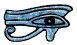# Introduction

We are so used to using a decimal system, a numbering system based upon ten, that most people never stop to think about it, let alone ask why, or what base ten means.

Man has been using a numbering system based upon ten for thousands of years. Why ten? Because Man first learned to count on his fingers!

Actually this isn’t strictly true: some people who went around bare-footed, such as the earliest shepherds, counted on their toes as well, so used base twenty. As they counted their sheep each night they would make a tally mark, or score, on their staff for every twenty, and a few traces of this earlier system still remain today. For example the French for ninety one is quatre vingt onze which in English literally means four score plus eleven.

Our present written numbers, 1, 2, 3 etc are usually known as Arabic numerals, although they actually originated in India. Today almost everybody, throughout the whole World, uses Arabic numerals and base ten. Some earlier ways of writing numbers, before Arabic numerals were introduced, are described on the Pages on Egyptian and Roman numerals.

Although Man has almost always used base ten for counting, there are advantages in using other bases for measuring, because we often need to divide something into three or four parts, or other fractions based upon these. The Ancient Babylonians used 12, 16, 24, 60 and 360 a lot because these numbers are easily divisible by multiples of 2, 3 and 4, and even today there are 60 minutes in an hour, 12 inches in a foot, 24 hours in a day, and 16 ounces in a pound (that is, an ounce is a quarter of a quarter of a pound), and a complete circle is 360 degrees.

Today for all sorts of reasons there is a lot of interest in bases other than ten, and this Page is intended to help you understand them. However, for reasons given below, it is intended mainly for adults and older students who are having trouble with understanding bases, not Year 5 children of average ability at Junior School being introduced to bases for the first time.

# More about base ten

To understand bases other than ten we do really need to understand about base ten.

We can write the number three thousand four hundred and fifty six as

3 × 1000 + 4 × 100 + 5 × 10 + 6 × 1

We can put these numbers into place-value columns, one column for the units, one for the tens, one for the hundreds and one for the thousands. The Ancient Egyptians wrote the units first, then the tens, then the hundreds and last the thousands, but today everyone does it the other way round.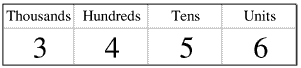If we have nine units and add one, we have ten, that is, one ten and no units, so we write a zero in the units column and a one in the tens column: although there are ten possible values for the number in the units column, the values go from 0 to 9 not 1 to 10. Similarly for the tens, hundreds and thousands columns.

This gives rise to the totally sterile argument which rears its head every ten years or so (but particularly at the end of the last millenium): did the “noughties” go from 2000 to 2009 or did they go from 2001 to 2010? Most of us are quite happy with the idea that if a set of books is in ten volumes they should be numbered 1 to 10: the first ten counting (natural) numbers are 1 to 10 not 0 to 9. But we can still choose to call the ten years 2000 to 2009 the noughties. It is a question of language not mathematics. For the record, the people who thought that the World would come to an end at the end of the first millennium were expecting it to happen at the end of the year DCCCCLXXXXVIIII not at the end of the year M.

This is discussed at some length on the Zero page.

In a decimal system there are 10 ways of filling the units column. We do not start filling the second column until the first column is full. So the second column is the tens column. Similarly there are 10 ways of filling the second column, so there are 10 × 10 ways of filling the first two columns. We start putting numbers into the third column only after we have filled the first two columns. So the third column is the ten squared column. Similarly the fourth column is the ten cubed column.

# A Note on powers

We can write 7 × 7 as 72 - we say this as “seven squared” or “seven to the power of two”. Similarly we can write 5 × 5 × 5 as 53 - we say this as “five cubed” or “five to the power of three”. Similarly 9 × 9 × 9 × 9 × 9 is 95; or “nine to the power of five”.

Similarly 178 = 17 × 17 × 17 × 17 × 17 × 17 × 17 × 17 or “seventeen to the power of eight” - do not try to multiply it out! This very simple idea leads to a whole new branch of mathematics. This is described on another Page of my Web Site.

35 × 34 = 3 × 3 × 3 × 3 × 3    ×     3 × 3 × 3 × 3   or  39.

Similarly by writing all the numbers out in full we can show that 118 × 1112 = 1120. This leads us to a simple Rule:

am × an = a(m + n)

where a, m and n are any numbers except a = 0

Using this Rule 64 × 61 = 65

But by writing the numbers out in full we can show that 64 × 6 = 65

This leads us to the conclusion that 61 = 6. In the same way we can also show that 91 = 9, so our second Rule is

a1 = a     (unless a = 0)

This may not be obvious at first sight but it is quite straightforward. The next part is not quite so straightforward but it is worth trying to master it.

Using the first Rule above we have 72 × 70 = 72.

Question: What number do I have to multiply 49 by to get the answer 49?

Slightly more difficult Question: What number do I have to multiply 34567847 by to get the answer 34567847?

Easy. 1. I thought you said the question was more difficult!

If 72 × 70 = 72 then 70 must be 1. Similarly if 94 × 90 = 94 then 90 must be 1. This leads us onto our third Rule

a0 = 1     (unless a = 0)

This Rule is very important in lots of different ways. Among other things it means that

1 = 100

10 = 101

100 = 102

1000 = 103

So we can write three thousand four hundred and fifty six as

3 × 103 + 4 × 102 + 5 × 101 + 6 × 100

and label the columns in the same way.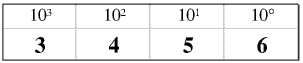This is the key to understanding bases other than ten. Unfortunately it is not the way most children at Junior Schools are taught about bases, even about base ten.

# Bases other than ten

We could choose to start with any base but I have chosen heptal, base 7, because seven is the largest prime number less than ten. Heptal might be useful for organisations working in days and weeks rather than days and months, which is more and more every year. If you understand heptal you can understand any base.

First remember or work out that (in decimal) 70 = 1, 71 = 7, 72 = 49, 73 = 343, 74 = 2401.

Then remember that in the same way that in decimal there are ten digits, going from 0 to 9 not 1 to 10, so in heptal there are seven digits, going from 0 to 6 - there is no 7 in a number written in heptal.

Finally remember the headings of the columns are powers of 7 not powers of 10.

Here are the first sixteen numbers in heptal, with their decimal equivalents.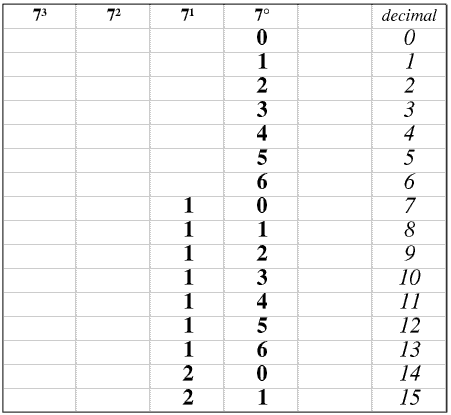We usually show a number written in heptal like this (3456)7. In place-value columns this would be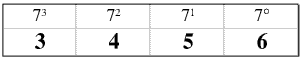If we take the heptal number (3456)7, in decimal it would be 3× 73 + 4 × 72 + 5 × 71 + 6 × 70 or 1266 - this is easy if you have a scientific calculator and know how to use it.

To convert the decimal number 5678 to heptal we proceed as follows:

2401 (74) goes into 5678 2 times remainder 876

343 (73) goes into 876 2 times remainder 190

49 (72) goes into 190 3 times remainder 43

7 (71) goes into 43 6 times remainder 1

So 5678 = 2 × 74 + 2 × 73 + 3 × 72 + 6 × 71 + 1 × 70.

Check this on your calculator if you don’t believe me. So (5678)10 = (22361)7.

There are some more worked examples on another Page of my web site.

# Binary

Even the World’s very latest and most powerful computers are incredibly stupid: they have to count on their fingers (digits). Mind you, they do have rather a lot of fingers and they can count quite quickly.

Each finger in the computer can be in one of two positions, up or down, so a computer uses a binary system, base 2. Each computer finger is called a binary digit, or bit. A bit can be 0, for finger down, or 1, for finger up. We can store numbers, or instructions, even pictures or sounds, as a bit pattern of 0s and 1s. Here are the first thirty three binary numbers in binary, and their decimal (and also hexadecimal - see below) equivalents.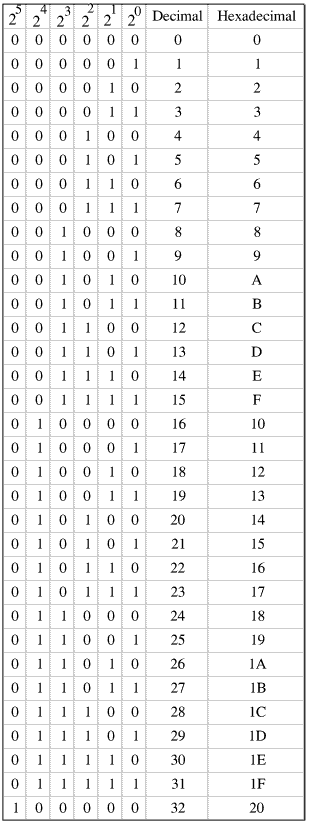Today when we use the term computer we usually mean a computing machine with four special properties.

• Electronic
• Digital
• Programmable
• Stored program

There have been and still are many computing machines with some but not all of these properties: the World’s first computer with all of them is usually, but wrongly, considered to be Colossus. This was built by British mathematicians for their work on code-breaking at Bletchley Park during the Second World War and was vital to the success of the Allied code-breaking operation, which shortened the War by at least two years. But a German called Konrad Zuse (1910 - 1995) had built a true computer before Colossus, and went on to develop others, but Nazi Germany saw no use for them and would not allow any more work on them (fortunately for the Allies!). Near the end of the War Zuse smuggled one of them into Switzerland and continued his work there.

The people who developed and used Colossus were very special people doing a very special job, and worked entirely in binary code and long bit patterns. But when computers began to be used by more ordinary people and for more ordinary things binary code and long bit patterns were rather clumsy, so people started arranging bit patterns into groups, as for example today we write five hundred and sixty five million two hundred and seventy two thousand nine hundred and fifty one as 565 272 951. Initially some people grouped the bits into patterns of 3. In 3 bits you can store 8 numbers (0 - 7) so this led to the use of octal (base 8), but others used patterns of 4. In four bits we can store sixteen numbers, and this leads us to the use of hexadecimal (base 16).

As computers became more powerful the bits were connected together inside them in different ways and the term byte (from bite-size) was introduced for the number of bits the computer could process (chew on) at a time. Today a byte is usually 8 or 16 or 24 or 32 or 48 or 64 bits, although other values have been and may still be used, depending on the way the processor is designed (its architecture). Where the computer’s bite-size is bigger than 8 bits the term word is often used instead of byte, and so it is often said that a byte is 8 bits.

Here is a table of all the powers of 2 from 20 to 240, with their decimal values.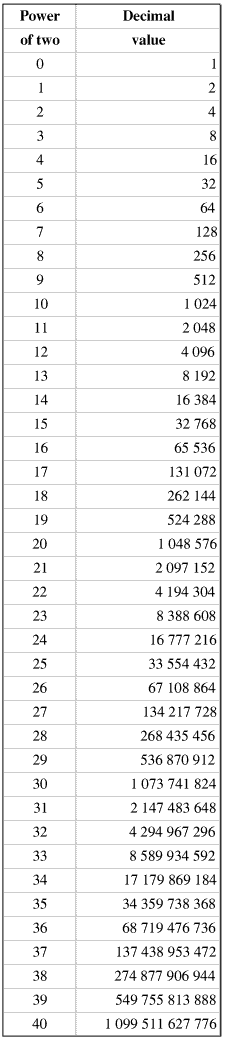It may be helpful for you to try to remember, or at any rate be able to recognise, all the powers of two up to two to the power of ten.

The terms kilobyte, megabyte, gigabyte and terrabyte are very commonly used. A kilobyte (kB) is used for 210 or 1024 bytes, not exactly a thousand bytes, a megabyte (MB) for 220 or approximately a million bytes, a gigabyte (GB) for 230 or approximately a thousand million (American billion) bytes and a terabyte (TB) for 240 or approximately a million million (American trillion) bytes.

Correctly the symbol k means a thousand, so the official, SI, name for 1024 bytes is a kibibyte, symbol KiB, for kilobinarybyte; similarly mebibyte, MiB, gibibyte, GiB, and tebibyte, TiB. Note that, unusually, kibibyte is KiB with a capital K - this anticipates the proposed change to capitalise all uses of k for kilo-, to bring it into line with M, G and T etc. Although these names were introduced in 2000 they are still not widely used.

If we have the binary number (110 1110 1011)2, in decimal it is

1×210 + 1×29 + 0×28 + 1×27 + 1×26 + 1×25 + 0×24 + 1×23 + 0×22 + 1×21 + 1×20

or 1024 + 512 + 128 + 64 + 32 + 8 + 2 + 1 or 1771.

To convert 1482 to binary we do

1482 - 1024 = 458   458 - 256 = 202   202 - 128 = 74   74 - 64 = 10   10 - 8 = 2

So 1482 = 1024 + 256 + 128 + 64 + 8 + 2

or 210 + 28 + 27 + 26 + 23 + 21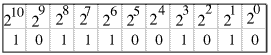So (1482)10 = (10111001010)2

Writing numbers in binary is very tedious and you are very likely to make a mistake. This is where hexadecimal comes in.

Hexadecimal (base 16) works exactly the same as decimal or heptal, except that the columns represent powers of 16. As 16 = 24, from the above Table we can see that 160 = 1, 161 = 16, 162 = 256 and 163 = 4096. There is one slight problem: we have to invent characters to represent the numbers with decimal values 10 to 15. Originally we used the capital letters A to F, although today some people use the small letters a to f. Here are the first few numbers in hexadecimal and decimal.Today hexadecimal is important because it is a much more convenient way of writing and working than binary, although computers themselves work only in binary. Hecadecimal numbers are often preceded by #. If when you are using your computer you come across a string of numbers and letters A to F (or a - f) it will almost certainly be a hexadecimal representation of a binary bit pattern. You may find hexadecimal in the HTML coding for the colour of an object on a web page, for example chocolate is #D2691E.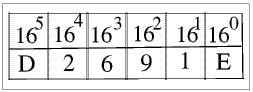To convert from hexadecimal to binary we substitute the four-bit pattern for each hexadecimal digit using the table above, and visa versa.

In binary #D2691E is 110100100110100100011110 Perhaps now you understand why we use hexadecimal....

This is 24 bits (numbered 0 - 23 of course), which is why we refer to it as 24 bit colour. From the table above you can see that using 24 bits we have a choice of more than eight million different colours, rather more than most of us can distinguish. For many purposes we do not need 24 bit colour, so often we use fewer bits and so fewer colours (for example GIFS rather than JPEGs). The more bits you use the longer it takes to render a page, so a 24 bit animated picture may appear very jerky unless you are using a much more powerful (and expensive!) graphics card than the sort usually fitted to most standard PCs and laptops.

There are some more worked examples of binary and hexadecimal on another Page.

If your printer starts printing whole lines of closely packed random characters, including lots of characters you may never have seen before, it is likely you are trying to print a data file, for example with filetype .exe or .iso, which is not intended to be printed.

Passwords and unlock codes which you have to type in are also often strings of numbers and letters, but they can contain any letters, not only A - F, and both upper-case and lower-case. This is for security reasons, to make them more difficult to remember or guess or copy down by looking over someone's shoulder, and to make it impossible for even a computer to try every possible combination of letters and numbers in less than several hours. Codes used for encrypting e-mails and internet banking etc work in a different way: the keys are hundreds, thousands or even tens of thousands of digits long and you do not have to type the key in, but there are so many combinations that it would take the world's most powerful computers hundreds of years to try them all. This is discussed on another Page.

# Sexagesimal

It is often said that the Ancient Babylonians used sexagesimal, base 60, from six times ten. Do not confuse sexagesimal with hexadecimal, six plus ten.

The Babylonians were very advanced mathematicians but they wrote in cuneiform and it is very difficult to explain their numbering system and mathematics without using cuneiform to write the numbers. Cuneiform is described on the Page on Roman Numerals (under construction).

The Babylonians did use sixty a lot, because it is divisible by 2, 3, 4, 5, 6 and 10, and also 12, 15, 20 and 30, but I am not certain that it is helpful, or even correct, for us to think that four thousand years ago they were using base 60 in the same way that we use base 10, or 7, or 2, or 16 today.

© Barry Gray, revised November 2014